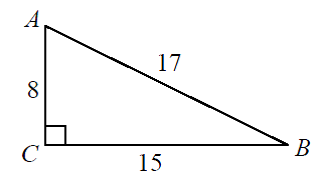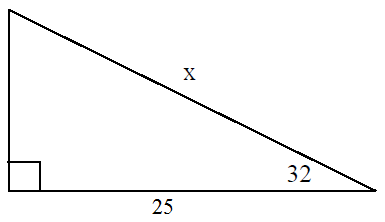# Usando coseno para calcular ángulos y lados (Cos = $\frac{a}{h}$ )

#### You’re one step closer to a better grade.

Learn with less effort by getting unlimited access, progress tracking and more.Learn More

### Introduction

#### Lessons

1. ¿Qué son el seno, coseno y tangente?

### Examples

#### Lessons

1. Determina los ángulos y lados usando la razón de Coseno:
1. Encuentra el ángulo $A$ y $B$ usando coseno:2. Encuentra el valor de "$x$" usando coseno:## Become a Member to Get More!

• #### Easily See Your ProgressWe track the progress you've made on a topic so you know what you've done. From the course view you can easily see what topics have what and the progress you've made on them. Fill the rings to completely master that section or mouse over the icon to see more details.

• #### Make Use of Our Learning Aids###### Practice Accuracy

See how well your practice sessions are going over time.

Stay on track with our daily recommendations.

• #### Earn Achievements as You LearnMake the most of your time as you use StudyPug to help you achieve your goals. Earn fun little badges the more you watch, practice, and use our service.

• #### Create and Customize Your AvatarPlay with our fun little avatar builder to create and customize your own avatar on StudyPug. Choose your face, eye colour, hair colour and style, and background. Unlock more options the more you use StudyPug.

###### Topic Basics
El coseno se define como la razón entre la medida del lado adyacente al ángulo en cuestión sobre la medida de la hipotenusa en un triángulo rectángulo.

## Trigonometric ratios

### cosine rule

The cosine rule tells us that when we have a right triangle, $cosine = \frac{a}{h}$. The "a" in this case stands for adjacent. The "h" stands for the hypotenuse, which can be found through the pythagorean theorem. In order to find cosine, all you'll need is the adjacent side and the hypotenuse.

### sine rule

When you come across sine, you can find the answer for it in a right triangle by taking the opposite side over the hypotenuse in the form of $sine = \frac{o}{h}$.

### tangent rule

For the tangent rule, when you have a right triangle, you can use the opposite over the adjacent sides of the triangle to find your ratio. This means that $tan = \frac{o}{a}$.

### SohCahToa

When you hear SohCahToa, it's not immediately obvious what it means. But it's actually an easier way for you to remember how to use sine, cosine, tangent that we just learned. These three are the main functions that you'll deal with in trigonometry problems.

Soh Cah Toa stands for:

It can help you find the length of a side of a right triangle as long as you have an angle $\theta$ and some info on the other sides of the triangle.

## Example problems

In this chapter, we're actually going to focus on the cosine rule. This means we'll only be working with the "$Cah$" portion of $SohCahToa$. Try out the following trig problems alongside us to learn how to solve questions using the cosine rule.

Question 1

Determine each cosine ratio using calculator

a) $\cos$ 50°

Simply put the number into your calculator and you should get $0.64$.

b) $\cos$ -50°

Simply put the number into your calculator and you should get $0.64$.

$\cos$ 50° and $\cos$ -50° both = $0.64$. Why?

This above ASTC chart helps you figure out which trig ratio is positive in which quadrant. $\cos$ 50° lies in quadrant I, where all the trig ratios are positive. $\cos$ -50° lies in quadrant 4, where cosine is positive. This is why we get $0.64$ for both $\cos$ 50° and $\cos$ -50°.

Question 2

Determine the angle to the nearest degree

Solution

a) $\cos \theta = 0.24$

Use the inverse cosine in the calculator which has a little $-1$ beside $cos$:

$\arccos (0.24) = 76$°

b) $\cos \theta = -0.45$

Use the inverse cosine in the calculator to find:

$\arccos (-0.45) = 117$°

Question 3

Determine the angles and sides using cosine

Solution:

a) Find angle $A$ and $B$:

$\cos \theta = \frac{adjacent}{hypotenuse}$

Angle $A$

$\cos A = \frac{8}{17}$

To solve it in your calculator

$\arccos \frac{8}{17} = 62$°

Angle $B$

$\cos B = \frac{15}{17}$

To solve it in your calculator

$\arccos \frac{15}{17} = 28$°

b) Find the value of "$x$" using cosine

$\cos \theta = \frac{adjacent}{hypotenuse}$
$\cos$ 32° = $\frac{25}{(x)}$
$x = \frac{25}{\cos 32}$
$x = 29$